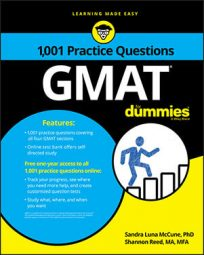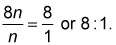##### GMATSome of the Problem Solving questions you'll encounter in the Quantitative section of the GMAT will involve basic math skills, where you'll need to work with fractions, decimals, ratios and proportions, percents, and exponents.

Problem Solving questions are multiple-choice questions with five answer choice options. You must select the one best answer choice.

## Practice questions

1. One plant grew 6 inches while a second plant grew 250 percent more than the first plant grew. How much, in inches, did the second plant grow?

A. 6 B. 8 C. 12 D. 15 E. 21

2. The number of squares is 4 times the number of circles, and the number of circles is twice the number of triangles. What is the ratio of the number of squares to the number of triangles?

A. 8:1 B. 4:1 C. 1:2 D. 1:4 E. 1:8

1. The correct answer is E.

The second plant grew 6 inches plus 250 percent of 6 inches, which is 6 in + 250% (6 in) = 6 in + 2.5 (6 in) + 15 in = 21 in.

2. The correct answer is A.

Let n = the number of triangles; then 2n = the number of circles; and 4 (2n) = 8n = the number of squares. The ratio of the number of squares to the number of triangles is